﻿• 价格透明
• 信息保密
• 进度掌控
• 售后无忧# OCR文本扫描 轮廓检测 透视变换

## 本项目和源代码来自唐宇迪opencv项目实战

### OCR文本识别

OCR （Optical Character Recognition，光学字符识别）是指电子设备（例如扫描仪或数码相机）检查纸上打印的字符，通过检测暗、亮的模式确定其形状，然后用字符识别方法将形状翻译成计算机文字的过程；即，针对印刷体字符，采用光学的方式将纸质文档中的文字转换成为黑白点阵的图像文件，并通过识别软件将图像中的文字转换成文本格式，供文字处理软件进一步编辑加工的技术。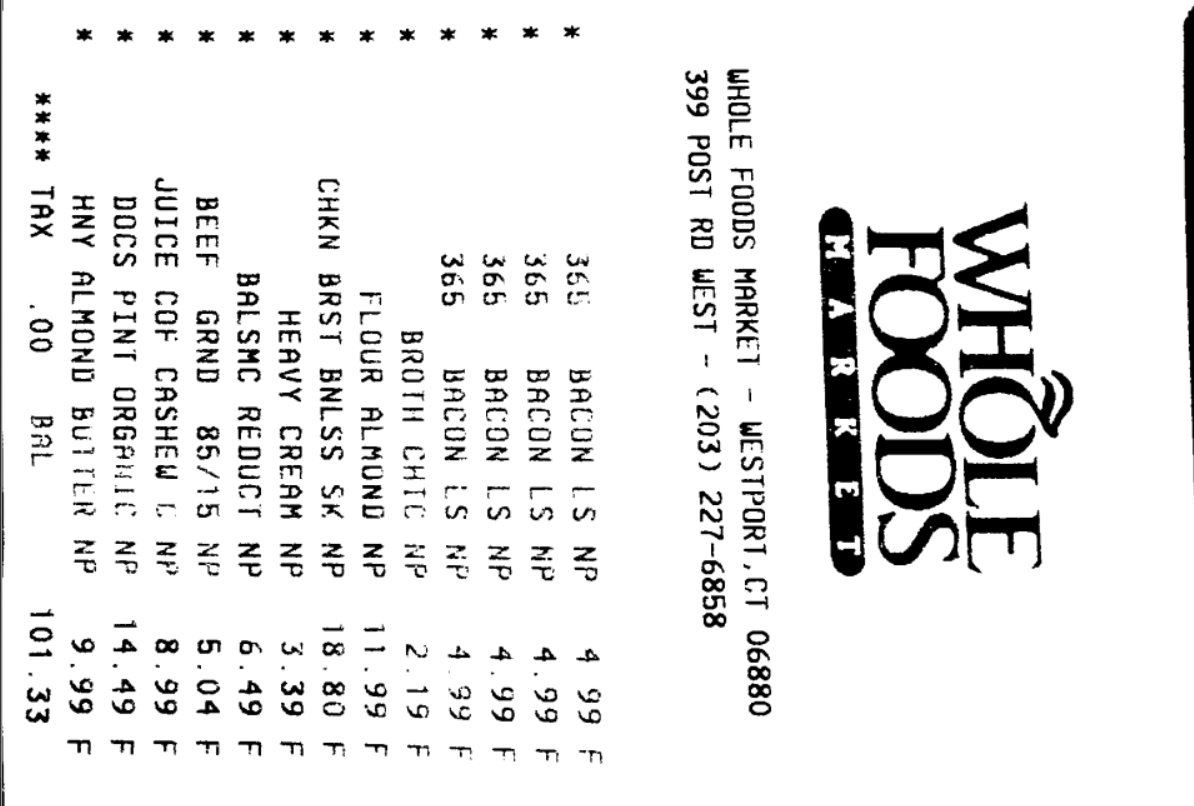## 算法步骤

### 1、导入图片

``````image = cv2.imread('receipt.jpg')
``````

### 2、图片与处理

#### resize 操作

resize函数的作用是按照原图像相同长宽比，当给定长（height）或者宽（width）时将原图resize成与原图像同比例的大小。

1 实验使用的图像多为手机拍摄的图片，图片大小至少为3500*4000，在imshow()，在屏幕显示并不能像是完整的图像，不利于观察。

#### Canny边缘检测步骤

1、canny边缘检测

1. ``````   使用高斯滤波器，以平滑图像，滤除噪声。
``````
2. ``````   计算图像中每个像素点的梯度强度和方向。
``````
3. ``````   应用非极大值（Non-Maximum Suppression）抑制，以消除边缘检测带来的杂散响应。
``````
4. ``````   应用双阈值（Double-Threshold）检测来确定真实的和潜在的边缘。
``````
5. ``````   通过抑制孤立的弱边缘最终完成边缘检测。
``````

1、高斯滤波器：通过高斯分布去除噪点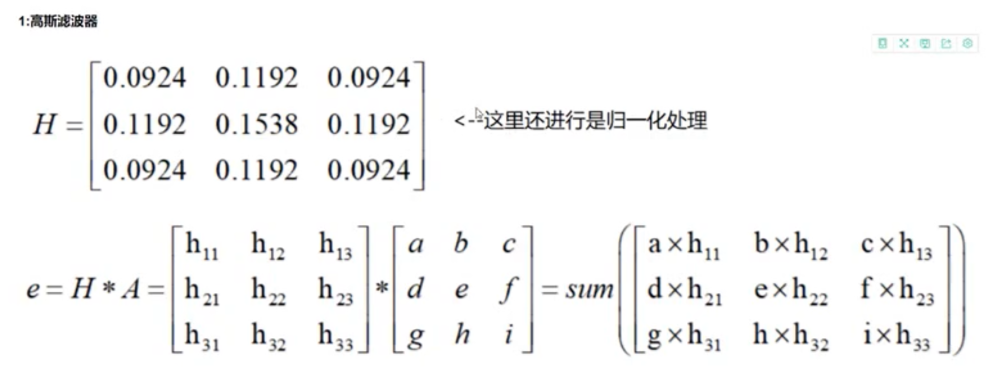2、计算Gx和Gy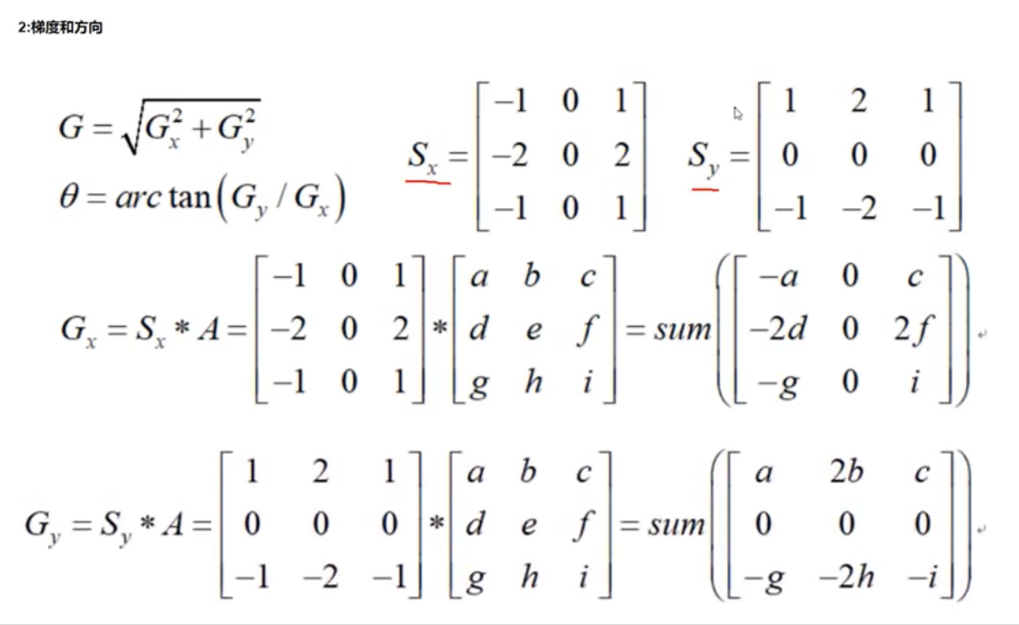3、非极大值抑制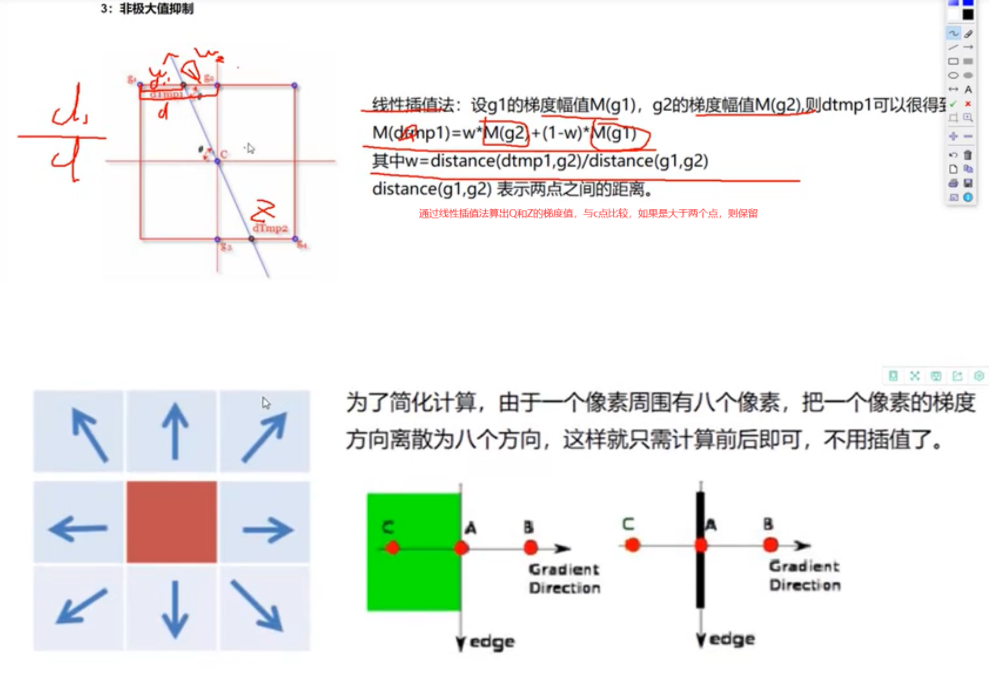4、双阈值检测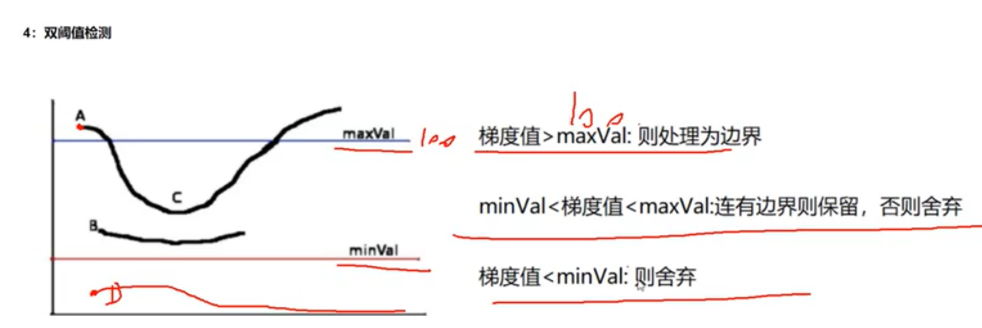``````import cv2 #opencv读取的格式是BGR
import numpy as np
import matplotlib.pyplot as plt#Matplotlib是RGB

def cv_show(name,img):
cv2.imshow(name,img)
#等待时间，毫秒级，0表示任意键终止
cv2.waitKey(0)#任意键终止
cv2.destroyAllWindows()

img1=cv2.Canny(img,120,250)
img2=cv2.Canny(img,50,100)
res=np.hstack((img1,img2))
cv_show('res',res)
``````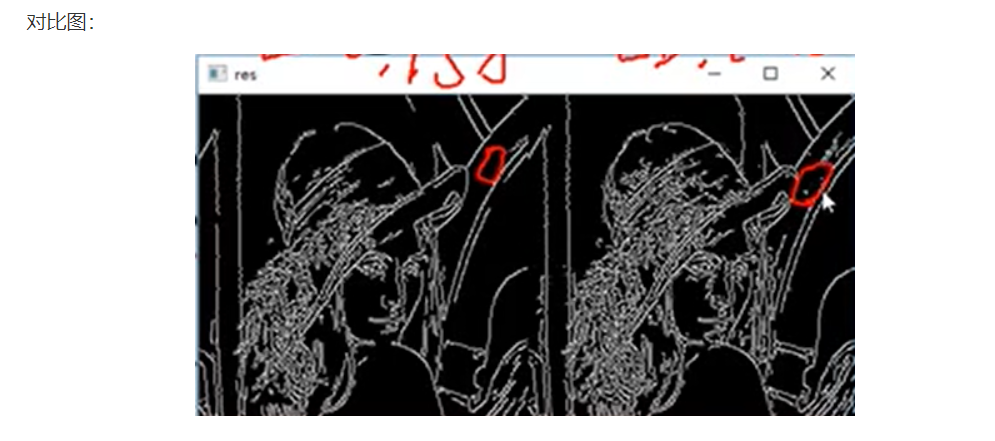#### Canny边缘检测之前需要先降噪----高斯滤波

GaussianBlur（src，ksize，sigmaX [，dst [，sigmaY [，borderType]]]）-> dst

Size ksize: 高斯内核大小，这个尺寸与前面两个滤波kernel尺寸不同，ksize.width和ksize.height可以不相同但是这两个值必须为正奇数，如果这两个值为0，他们的值将由sigma计算。
double sigmaX: 高斯核函数在X方向上的标准偏差
double sigmaY: 高斯核函数在Y方向上的标准偏差，如果sigmaY是0，则函数会自动将sigmaY的值设置为与sigmaX相同的值，如果sigmaX和sigmaY都是0，这两个值将由ksize.width和ksize.height计算而来。

#### Canny边缘检测函数

canny = cv2.Canny(gauss, 75, 200)

``````# 预处理
gray = cv2.cvtColor(image, cv2.COLOR_BGR2GRAY)#灰度
gray = cv2.GaussianBlur(gray, (5, 5), 0)#高斯滤波
edged = cv2.Canny(gray, 75, 200)#边缘检测：双阈值检测，75为mix，200为max。让边缘都成白色，其余为黑色

# 展示预处理结果
print("STEP 1: 边缘检测")
cv_show("Image", image)
cv_show("Edged", edged)
``````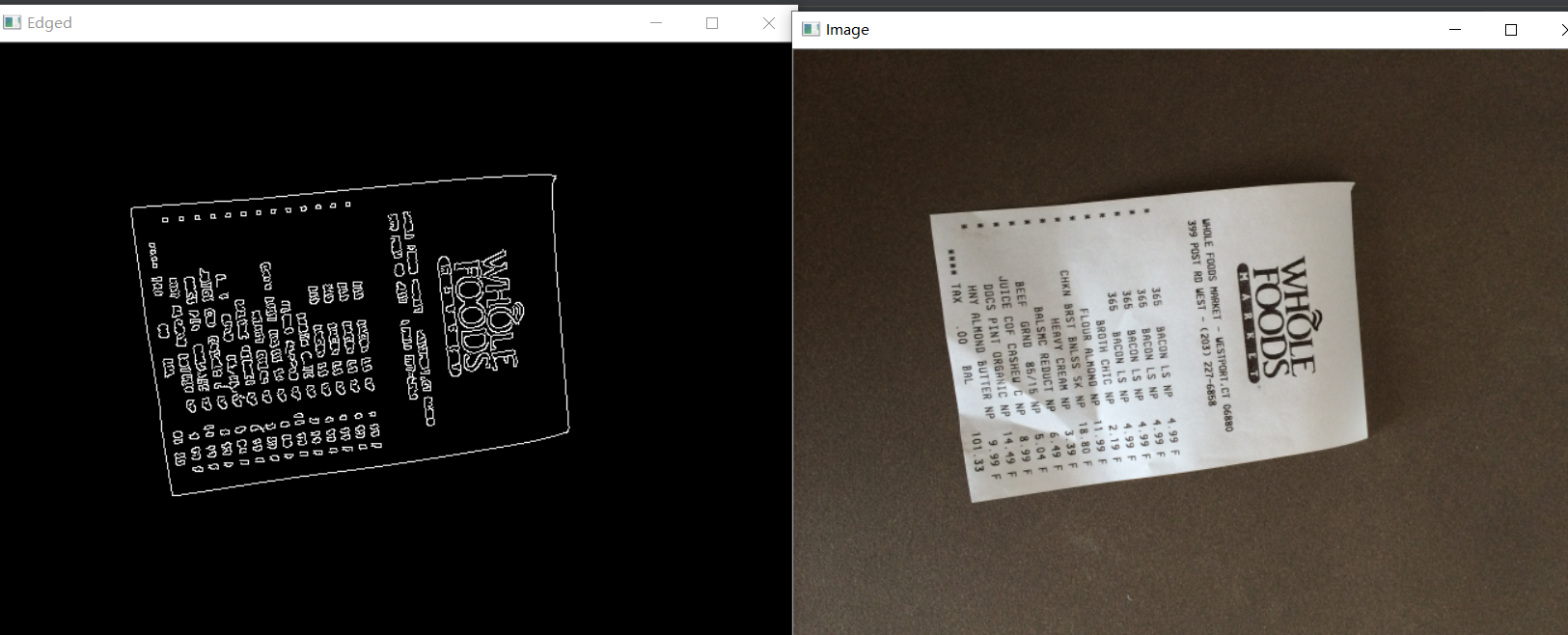#### 轮廓检测

cv2.findContours(img, mode, methord)

MODE注释
RETR_EXTERNAL只检测最外面的轮廓
RETR_LIST检索所有轮廓，并将其保存到一条链表中
RETR_CCOMP检索所有轮廓，并将他们组织为两层 ，顶层是各部分的外界边界，第二层是空洞的边界
RETR_TREE检测所有轮廓，并重构嵌套轮廓的整个层次

#### 轮廓近似

peri = cv2.arcLength(c, True)

approx = cv2.approxPolyDP(c, 0.02*peri, True)
True表示轮廓是封闭的

#### 透视变换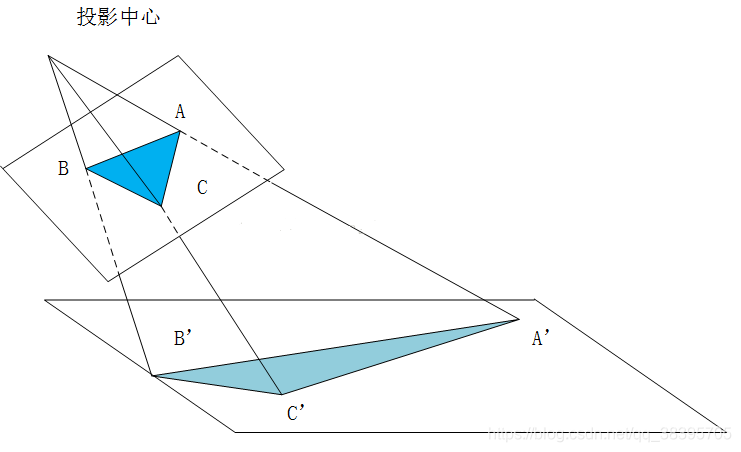#### 1、cv2.getPerspectiveTransform(src, dst)

``````src：源图像中待测矩形的四点坐标
sdt：目标图像中矩形的四点坐标
``````

#### 2、cv2.warpPerspective(src, M, dsize[, dst[, flags[, borderMode[, borderValue]]]])

src：输入图像
M：变换矩阵
dsize：目标图像shape
flags：插值方式，interpolation方法INTER_LINEAR或INTER_NEAREST
borderMode：边界补偿方式，BORDER_CONSTANT or BORDER_REPLICATE
borderValue：边界补偿大小，常值，默认为0

#### 3、cv2.perspectiveTransform(src, m[, dst])

src：输入的2通道或者3通道的图片
m：变换矩阵

#### 4、区别

warpPerspective适用于图像。perspectiveTransform适用于一组点。

##### 自定义函数 order_points()
``````def order_points(pts):
# 一共4个坐标点
rect = np.zeros((4, 2), dtype = "float32")

# 按顺序找到对应坐标0123分别是 左上，右上，右下，左下
# 计算左上，右下
s = pts.sum(axis = 1)
rect = pts[np.argmin(s)]
rect = pts[np.argmax(s)]

# 计算右上和左下
diff = np.diff(pts, axis = 1)
rect = pts[np.argmin(diff)]
rect = pts[np.argmax(diff)]

return rect
``````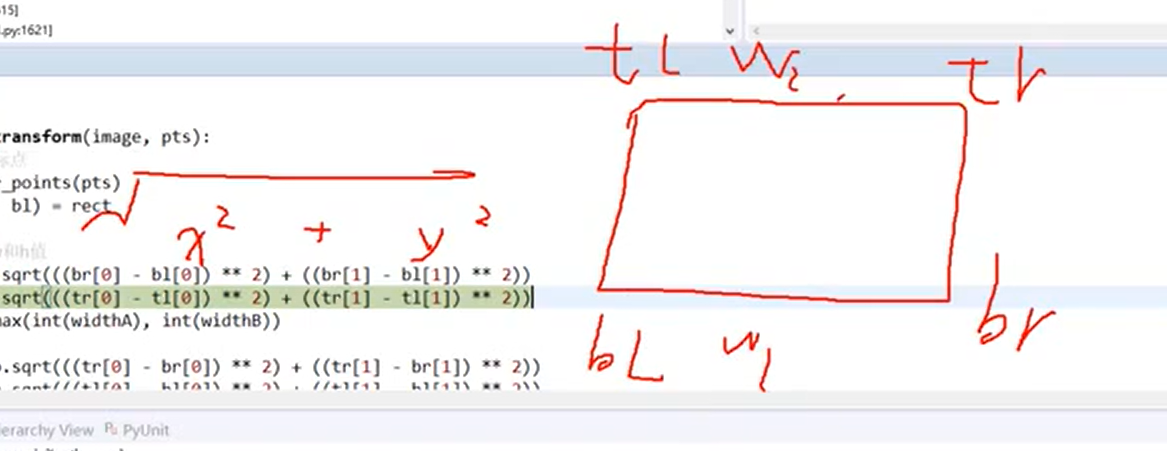#### 自定义函数 def four_point_transform()

``````def four_point_transform(image, pts):
# 获取输入坐标点
rect = order_points(pts)
(tl, tr, br, bl) = rect

# 计算输入的w和h值
widthA = np.sqrt(((br - bl) ** 2) + ((br - bl) ** 2))   #使用的公式s = ((x2-x1)^2  + (y2-y1))^2)^1/2 算出两边宽度
widthB = np.sqrt(((tr - tl) ** 2) + ((tr - tl) ** 2))
maxWidth = max(int(widthA), int(widthB))

heightA = np.sqrt(((tr - br) ** 2) + ((tr - br) ** 2))
heightB = np.sqrt(((tl - bl) ** 2) + ((tl - bl) ** 2))
maxHeight = max(int(heightA), int(heightB))

# 变换后对应坐标位置
dst = np.array([
[0, 0],
[maxWidth - 1, 0],
[maxWidth - 1, maxHeight - 1],
[0, maxHeight - 1]], dtype = "float32")

# 计算变换矩阵
M = cv2.getPerspectiveTransform(rect, dst)
warped = cv2.warpPerspective(image, M, (maxWidth, maxHeight))

# 返回变换后结果
return warped
``````

dst对应的是与原图像中轮廓大小相同，只是进行了坐标变换的图像。左上角坐标点为（0，0），图像的长、宽分别为四边形轮廓长、宽最大值的四个定位点。将原图像中卡片的轮廓抠出来，变换了坐标。

``````M = cv2.getPerspectiveTransform(rect, dst)
warped = cv2.warpPerspective(image, M, (maxWidth, maxHeight))
``````

``````warped = four_point_transform(image, screenCnt.reshape(4, 2))
warped = four_point_transform(orig, screenCnt.reshape(4, 2)*ratio)
``````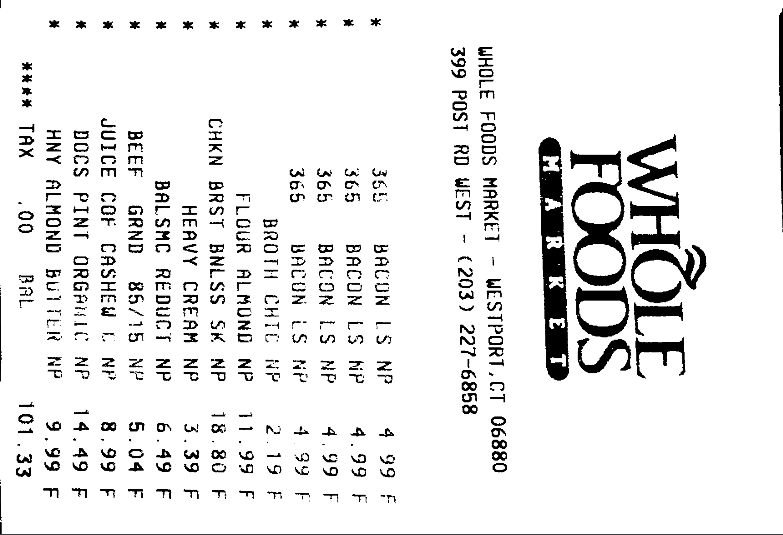#### 调用four_point_transform函数

warped = four_point_transform(orig, screenCnt.reshape(4, 2)*ratio)

#### 对于 M = cv2.getPerspectiveTransform(rect, dst)

3的矩阵中，有8个未知数，就有8个方程，最后一位是1，一组坐标可以确定2个方程，因此需要4组坐标，即一个矩形的四个角的坐标。这个函数就是帮我们算矩阵m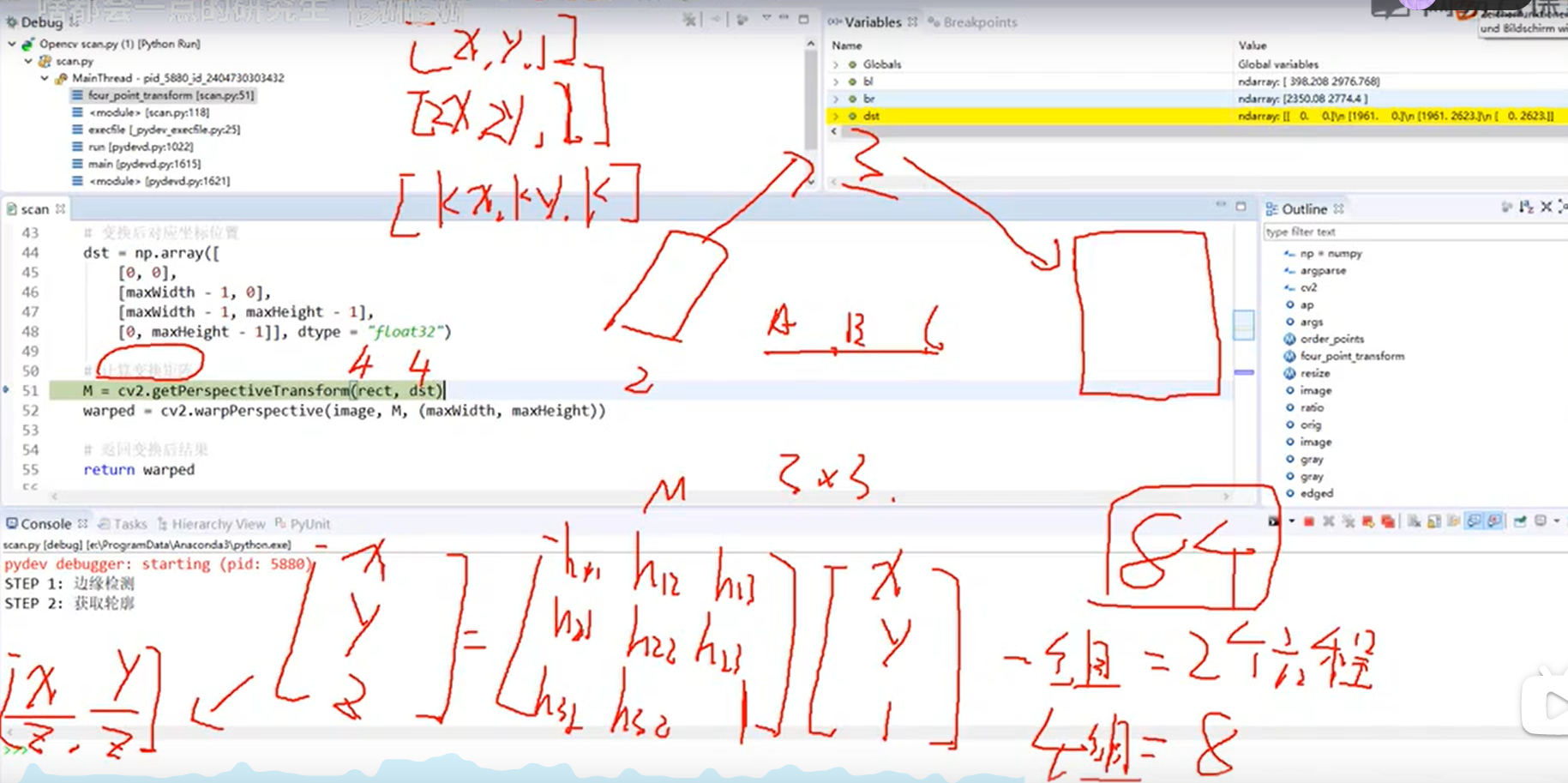## 3 调用pytesseract进行OCR文本识别

### 接着在test.py中 import pytesseract 进行OCR识别

``````# https://digi.bib.uni-mannheim.de/tesseract/
# 配置环境变量如E:\Program Files (x86)\Tesseract-OCR
# tesseract -v进行测试
# tesseract XXX.png 得到结果
# pip install pytesseract
# anaconda lib site-packges pytesseract pytesseract.py
# tesseract_cmd 修改为绝对路径即可
from PIL import Image
import pytesseract
import cv2
import os

preprocess = 'blur' #thresh

gray = cv2.cvtColor(image, cv2.COLOR_BGR2GRAY)

if preprocess == "thresh":
gray = cv2.threshold(gray, 0, 255,cv2.THRESH_BINARY | cv2.THRESH_OTSU)

if preprocess == "blur":
gray = cv2.medianBlur(gray, 3)

filename = "{}.png".format(os.getpid())
cv2.imwrite(filename, gray)

text = pytesseract.image_to_string(Image.open(filename))
print(text)
os.remove(filename)

cv2.imshow("Image", image)
cv2.imshow("Output", gray)
cv2.waitKey(0)

``````

gray = cv2.medianBlur(gray, 3)

pytesseract.image_to_string(Image.open(filename))### 低价透明### 金牌服务### 信息保密